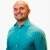# SAT Prep How to determine the percentage of a group of studentsThis lesson will look at an example problem involving percentage. This lesson will give an explanation of how to determine the percentage of a group of students with a step by step look to better explain... This lesson will look at an example problem involving percentage. This lesson will give an explanation of how to determine the percentage of a group of students with a step by step look to better explain the concept.
More... Collapse
8 Views